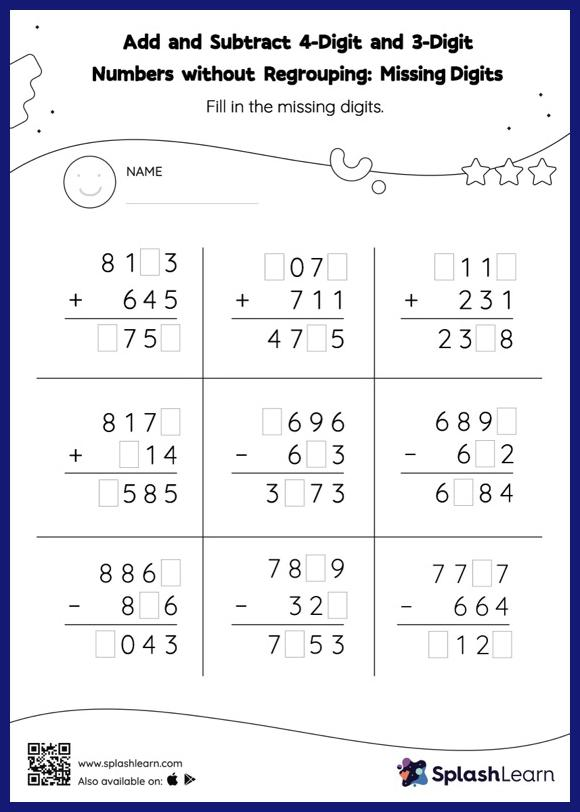# Add and Subtract 4-Digit and 3-Digit Numbers without Regrouping: Missing Digits Worksheet

Home > Add and Subtract 4-Digit and 3-Digit Numbers without Regrouping: Missing DigitsChildren use their math skills to complete this add and subtract 4-digit and 3-digit numbers without regrouping worksheet. In this worksheet, students must find the missing number using the relationship between addition and subtraction. To solve add and subtract 4-digit and 3-digit numbers without regrouping worksheet, they do not need to regroup the numbers.